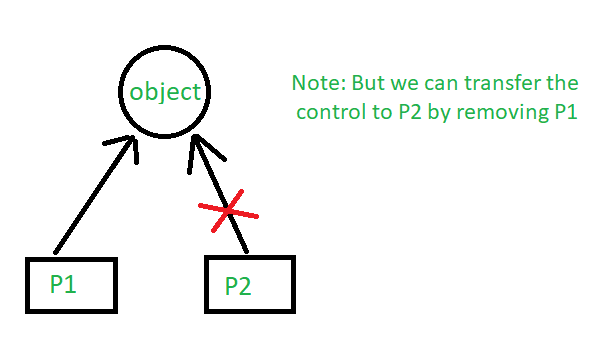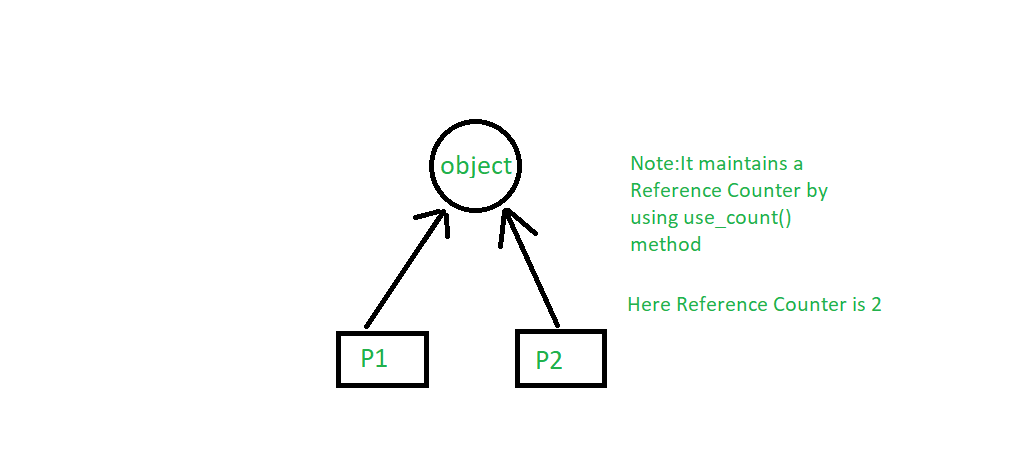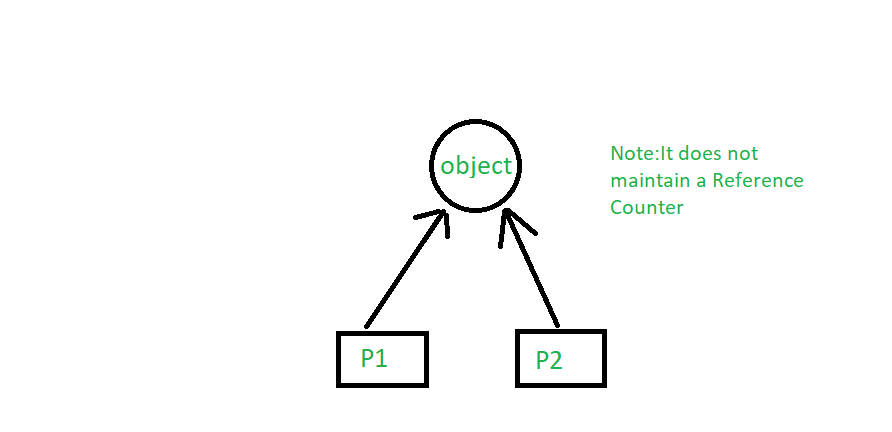Open in App
Not now

# Smart Pointers in C++ and How to Use Them

• Difficulty Level : Medium
• Last Updated : 01 Dec, 2021

In this article, we will be discussing smart pointers in C++. What are Smart Pointers, why, and how to use them properly?

Pointers are used for accessing the resources which are external to the program – like heap memory. So, for accessing the heap memory (if anything is created inside heap memory), pointers are used. When accessing any external resource we just use a copy of the resource. If we make any change to it, we just change it in the copied version. But, if we use a pointer to the resource, we’ll be able to change the original resource.

## Problems with Normal Pointers

Take a look at the code below.

## C++

 `#include ` `using` `namespace` `std;`   `class` `Rectangle {` `private``:` `    ``int` `length;` `    ``int` `breadth;` `};`   `void` `fun()` `{` `    ``// By taking a pointer p and` `    ``// dynamically creating object` `    ``// of class rectangle` `    ``Rectangle* p = ``new` `Rectangle();` `}`   `int` `main()` `{` `    ``// Infinite Loop` `    ``while` `(1) {` `        ``fun();` `    ``}` `}`

In function fun, it creates a pointer that is pointing to the Rectangle object. The object Rectangle contains two integers, length and breadth. When the function fun ends, p will be destroyed as it is a local variable. But, the memory it consumed won’t be deallocated because we forgot to use delete p; at the end of the function. That means the memory won’t be free to be used by other resources. But, we don’t need the variable anymore, but we need the memory.

In function main, fun is called in an infinite loop. That means it’ll keep creating p. It’ll allocate more and more memory but won’t free them as we didn’t deallocate it. The memory that’s wasted can’t be used again. Which is a memory leak. The entire heap memory may become useless for this reason. C++11 comes up with a solution to this problem, Smart Pointer.

## Introduction of Smart Pointers

As we’ve known unconsciously not deallocating a pointer causes a memory leak that may lead to crash of the program. Languages Java, C# has Garbage Collection Mechanisms to smartly deallocate unused memory to be used again. The programmer doesn’t have to worry about any memory leak. C++11 comes up with its own mechanism that’s Smart Pointer. When the object is destroyed it frees the memory as well. So, we don’t need to delete it as Smart Pointer does will handle it.

A Smart Pointer is a wrapper class over a pointer with an operator like * and -> overloaded. The objects of the smart pointer class look like normal pointers. But, unlike Normal Pointers it can deallocate and free destroyed object memory.

The idea is to take a class with a pointer, destructor and overloaded operators like * and ->. Since the destructor is automatically called when an object goes out of scope, the dynamically allocated memory would automatically be deleted (or reference count can be decremented). Consider the following simple SmartPtr class.

## C++

 `#include ` `using` `namespace` `std;`   `class` `SmartPtr {` `    ``int``* ptr; ``// Actual pointer` `public``:` `    ``// Constructor: Refer https:// www.geeksforgeeks.org/g-fact-93/` `    ``// for use of explicit keyword` `    ``explicit` `SmartPtr(``int``* p = NULL) { ptr = p; }`   `    ``// Destructor` `    ``~SmartPtr() { ``delete` `(ptr); }`   `    ``// Overloading dereferencing operator` `    ``int``& operator*() { ``return` `*ptr; }` `};`   `int` `main()` `{` `    ``SmartPtr ptr(``new` `int``());` `    ``*ptr = 20;` `    ``cout << *ptr;`   `    ``// We don't need to call delete ptr: when the object` `    ``// ptr goes out of scope, the destructor for it is automatically` `    ``// called and destructor does delete ptr.`   `    ``return` `0;` `}`

Output:

`20`

This only works for int. So, we’ll have to create Smart Pointer for every object? No, there’s a solution, Template. In the code below as you can see T can be of any type. Read more about Template here.

## C++

 `#include ` `using` `namespace` `std;`   `// A generic smart pointer class` `template` `<``class` `T>` `class` `SmartPtr {` `    ``T* ptr; ``// Actual pointer` `public``:` `    ``// Constructor` `    ``explicit` `SmartPtr(T* p = NULL) { ptr = p; }`   `    ``// Destructor` `    ``~SmartPtr() { ``delete` `(ptr); }`   `    ``// Overloading dereferencing operator` `    ``T& operator*() { ``return` `*ptr; }`   `    ``// Overloading arrow operator so that` `    ``// members of T can be accessed` `    ``// like a pointer (useful if T represents` `    ``// a class or struct or union type)` `    ``T* operator->() { ``return` `ptr; }` `};`   `int` `main()` `{` `    ``SmartPtr<``int``> ptr(``new` `int``());` `    ``*ptr = 20;` `    ``cout << *ptr;` `    ``return` `0;` `}`

Output:

`20`

Note: Smart pointers are also useful in the management of resources, such as file handles or network sockets.

## Types of Smart Pointers

### 1. unique_ptr

unique_ptr stores one pointer only. We can assign a different object by removing the current object from the pointer. Notice the code below. First, the unique_pointer is pointing to P1. But, then we remove P1 and assign P2 so the pointer now points to P2.## C++14

 `#include ` `using` `namespace` `std;` `#include `   `class` `Rectangle {` `    ``int` `length;` `    ``int` `breadth;`   `public``:` `    ``Rectangle(``int` `l, ``int` `b){` `        ``length = l;` `        ``breadth = b;` `    ``}`   `    ``int` `area(){` `        ``return` `length * breadth;` `    ``}` `};`   `int` `main(){`   `    ``unique_ptr P1(``new` `Rectangle(10, 5));` `    ``cout << P1->area() << endl; ``// This'll print 50`   `    ``// unique_ptr P2(P1);` `    ``unique_ptr P2;` `    ``P2 = move(P1);`   `    ``// This'll print 50` `    ``cout << P2->area() << endl;`   `    ``// cout<area()<

Output:

```50
50```

### 2. shared_ptr

By using shared_ptr more than one pointer can point to this one object at a time and it’ll maintain a Reference Counter using use_count() method.## C++14

 `#include ` `using` `namespace` `std;` `#include `   `class` `Rectangle {` `    ``int` `length;` `    ``int` `breadth;`   `public``:` `    ``Rectangle(``int` `l, ``int` `b)` `    ``{` `        ``length = l;` `        ``breadth = b;` `    ``}`   `    ``int` `area()` `    ``{` `        ``return` `length * breadth;` `    ``}` `};`   `int` `main()` `{`   `    ``shared_ptr P1(``new` `Rectangle(10, 5));` `    ``// This'll print 50` `    ``cout << P1->area() << endl;`   `    ``shared_ptr P2;` `    ``P2 = P1;`   `    ``// This'll print 50` `    ``cout << P2->area() << endl;`   `    ``// This'll now not give an error,` `    ``cout << P1->area() << endl;`   `    ``// This'll also print 50 now` `    ``// This'll print 2 as Reference Counter is 2` `    ``cout << P1.use_count() << endl;` `    ``return` `0;` `}`

Output:

```50
50
50
2```

### 3. weak_ptr

It’s much more similar to shared_ptr except it’ll not maintain a Reference Counter. In this case, a pointer will not have a stronghold on the object. The reason is if suppose pointers are holding the object and requesting for other objects then they may form a Deadlock.C++ libraries provide implementations of smart pointers in the form of auto_ptr, unique_ptr, shared_ptr and weak_ptr

References:
http://en.wikipedia.org/wiki/Smart_pointer